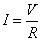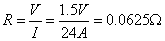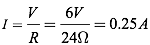# Ohm's Law

If resistance opposes current flow, and potential difference promotes current flow, it only makes sense that these quantities must somehow be related. George Ohm studied and quantified these relationships for conductors and resistors in a famous formula now known as Ohm's Law:Ohm's Law may make more qualitative sense if we re-arrange it slightly:Now it's easy to see that the current flowing through a conductor or resistor (in amps) is equal to the potential difference across the object (in volts) divided by the resistance of the object (in ohms). If you want a large current to flow, you would require a large potential difference (such as a large battery), and/or a very small resistance.

Question: The current in a wire is 24 amperes when connected to a 1.5 volt battery. Find the resistance of the wire.

Answer:Question: In a simple electric circuit, a 24-ohm resistor is connected across a 6-volt battery. What is the current in the circuit?

Answer:Note: Ohm's Law isn't truly a law of physics -- not all materials obey this relationship. It is, however, a very useful empirical relationship that accurately describes key electrical characteristics of conductors and resistors. One way to test if a material is ohmic (if it follows Ohm's Law) is to graph the voltage vs. current flow through the material. If the material obeys Ohm's Law, you'll get a linear relationship, and the slope of the line is equal to the material's resistance.

Question: The graph below represents the relationship between the potential difference (V) across a resistor and the current (I) through the resistor.Through which entire interval does the resistor obey Ohm’s Law?

1. AB
2. BC
3. CD